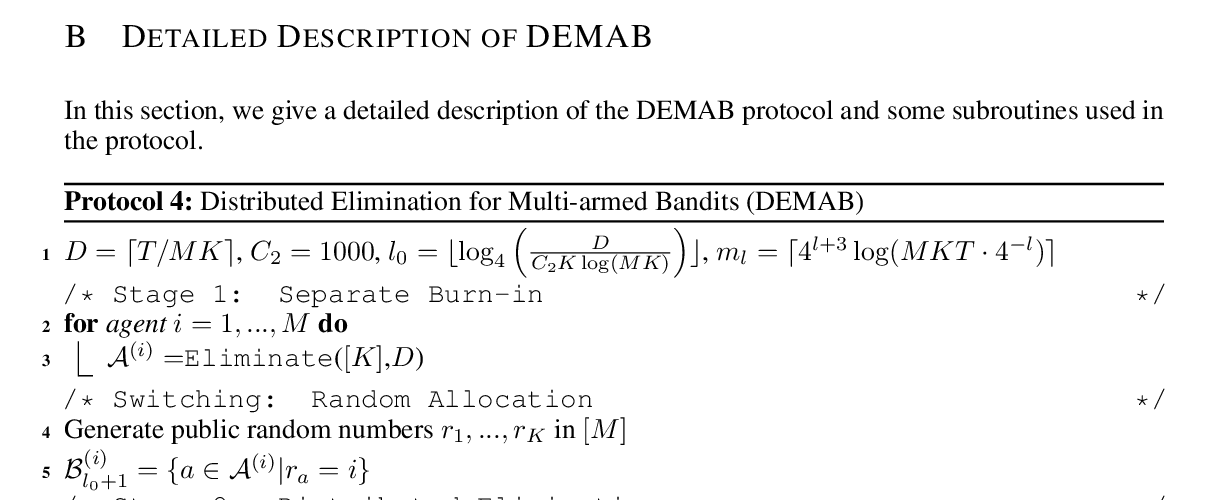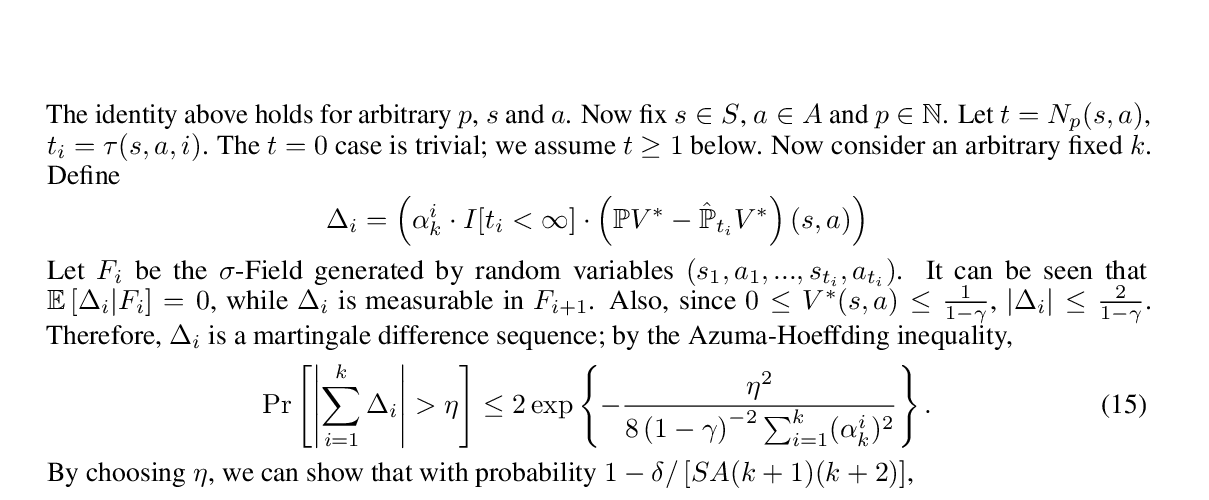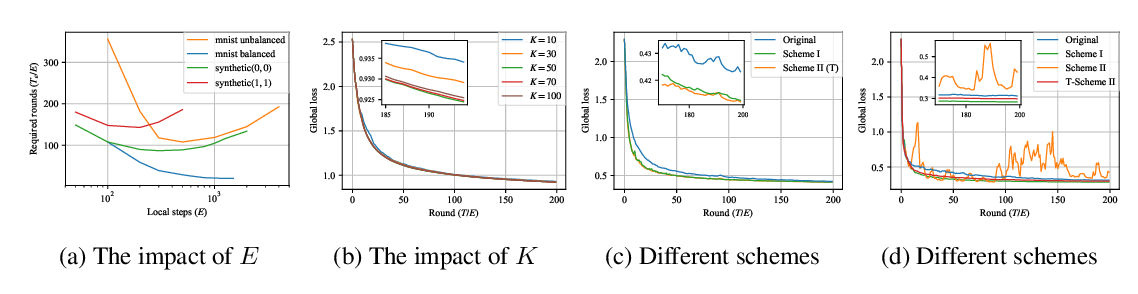### Guanghui Wang, Shiyin Lu, Quan Cheng, Wei-wei Tu, Lijun Zhang

Abstract: The Adam algorithm has become extremely popular for large-scale machine learning. Under convexity condition, it has been proved to enjoy a data-dependent $O(\sqrt{T})$ regret bound where $T$ is the time horizon. However, whether strong convexity can be utilized to further improve the performance remains an open problem. In this paper, we give an affirmative answer by developing a variant of Adam (referred to as SAdam) which achieves a data-dependent $O(\log T)$ regret bound for strongly convex functions. The essential idea is to maintain a faster decaying yet under controlled step size for exploiting strong convexity. In addition, under a special configuration of hyperparameters, our SAdam reduces to SC-RMSprop, a recently proposed variant of RMSprop for strongly convex functions, for which we provide the first data-dependent logarithmic regret bound. Empirical results on optimizing strongly convex functions and training deep networks demonstrate the effectiveness of our method.

## Similar Papers

##### Distributed Bandit Learning: Near-Optimal Regret with Efficient Communication
###### Yuanhao Wang, Jiachen Hu, Xiaoyu Chen, Liwei Wang,##### Q-learning with UCB Exploration is Sample Efficient for Infinite-Horizon MDP
###### Yuanhao Wang, Kefan Dong, Xiaoyu Chen, Liwei Wang,##### On the Convergence of FedAvg on Non-IID Data
###### Xiang Li, Kaixuan Huang, Wenhao Yang, Shusen Wang, Zhihua Zhang,